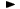ADVFN HomeHelpFinancialsKey FiguresNet Debt

# Net Debt

Net Debt, is defined as total liabilities minus cash & equivalents. The formula is the following and the figures are expressed in millions:

= total liabilities - cash & equivalents